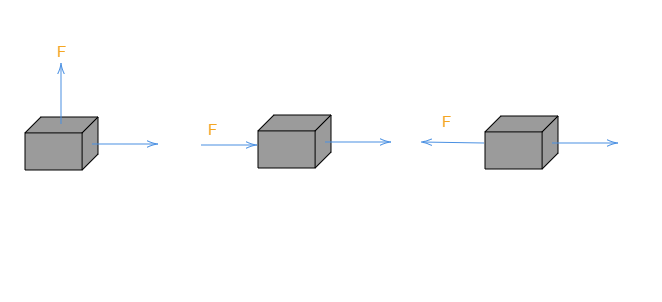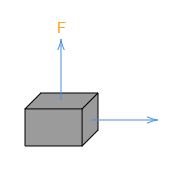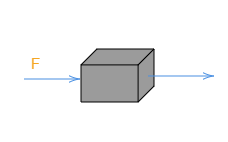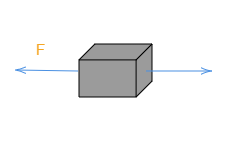# In each of the following a force, $F$ is acting on an object of mass, $m$. The direction of displacement is from west to east shown by the longer arrow. Observe the diagrams carefully and state whether the work done by the force is negative, positive or zero."

In 1st case:We know the formula for the work done, $\boxed{W=Fd\cos\theta}$

Here, $W\rightarrow$work done

$F\rightarrow$force

$d\rightarrow$displacement

$\theta\rightarrow$ angle between the direction of the applied force and displacement

In 1st case, the directions of the applied force and the displacement are perpendicular to each other.

So, $\theta=90^{\circ}$

So, $W=F\times d\times \cos90^{\circ}$

Or $W=Fd\times 0$                           [because $\cos90^{\circ}=0$]

So, the work done in 1st case is zero.

In the 2nd case:The direction of the applied force and the displacement are the same.

So, $\theta=0^{\circ}$

So, work done, $W=Fd\times \cos0^{\circ}$

Or $W=Fd\times 1$                    [Because $\cos0^{\circ}=1$]

Or $W=Fd$

So, the work done is positive in case 2nd.

In the case of 3rd:The direction of the applied force and the displacement are just opposite.

So, $\theta=180^{\circ}$

So, the work done, $W=Fd\cos180^{\circ}$

Or $W=Fd(-1)$                         [Because $\cos180^{\circ}=-1$]

Or $W=-Fd$

So, the work done is negative in case 3rd.